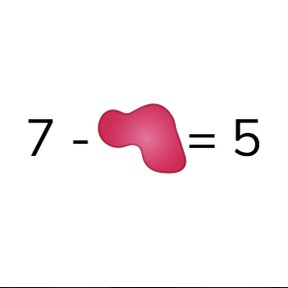Fill in the blank problems + - to 10

# Fill in the blank problems + - to 10

Solve fill in the blank math problems with addition & subtraction with numbers t8,000 schools use Gynzy92,000 teachers use Gynzy1,600,000 students use Gynzy

## General

Students learn to add and subtract in fill in the blank math problems up to 10.

## Standards

CCSS.Math.Content.K.OA.A

## Learning objective

Students will be able to add and subtract up to 10 in fill in the blank problems.

## Introduction

The interactive whiteboard has a number of addition and subtraction problems. Ask students to solve these problems and to write down their answers on a mini whiteboard or a sheet of paper. Discuss the answers and ask students to explain their strategy.

## Instruction

Check that students are able to solve fill in the blank math problems with addition & subtraction with numbers to 10 by asking the following questions:
- How can you easily determine which number belongs in the empty space of a math problem?
- What kind of math problem do you make to solve 4 + ... = 9?
- What kind of math problem do you make to solve ... - 6 = 7?

## Quiz

Students are first asked to fill in the blank with addition problems. Then they are given subtraction problems. They are also asked to determine if the problem should be completed with a plus or minus sign.

## Closing

Remind students that it is important to be able to determine which number fills in the blank and ask students how they can figure out which number completes a given math problem. The interactive whiteboard then has a collection of math problems which got paint splatters over the problems! Ask students to figure out which numbers complete the given math problems. You may have them work individually or in pairs. Make sure to have them explain their thinking.

## Teaching tips

Students who have difficulty with this learning goal can be supported by making use of a number line. They can count in steps from the given number to the total or to the difference.

### The online teaching platform for interactive whiteboards and displays in schools

• Save time building lessons

• Manage the classroom more efficiently

• Increase student engagement